Q-Chem 5.0 User’s Manual

# B.6 Fundamental ERI

The fundamental ERI  and the basis of all ERI algorithms is usually represented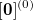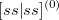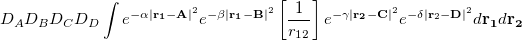(B.3)

which can be reduced to a one-dimensional integral of the form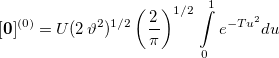(B.4)

and can be efficiently computed using a modified Chebyshev interpolation scheme . Equation (B.4) can also be adapted for the general case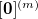integrals required for most calculations. Following the fundamental ERI, building up to the full bra-ket ERI (or intermediary matrix elements, see later) are the problems of angular momentum and contraction.

Note: Square brackets denote primitive integrals and parentheses denote fully-contracted integrals.